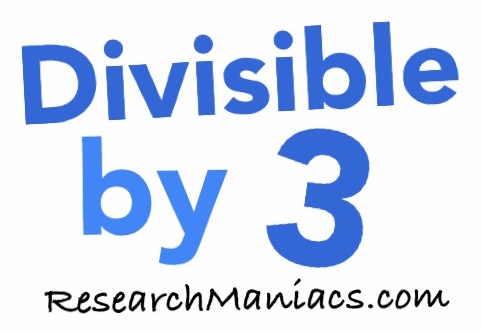Is 99 divisible by 3?

 Is 99 divisible by 3? In other words, if you divide 99 by 3, will you get a whole number with no remainder? Of course, you could use a calculator to find out if 99 is divisible by 3, but what fun would that be? To find out if 99 is divisible by 3, we will add up the digits that make 99 as follows: 9 + 9 = 18 We know that if the sum of the numbers that make up 99 is divisible by 3, then 99 is divisible by 3. Since the sum of the digits in 99 is divisible by 3, 99 is also divisible by 3. Thus, the answer to the question "Is 99 divisible by 3?" is as follows: Yes Note: If you divide 99 by 3, you get 33 which is a whole number. Is 100 divisible by 3? Can you do it on your own now? If not, go here for the next number on our list.Divisible by 3? Do you need to check another number? Enter a number below to see if it is divisible by 3.